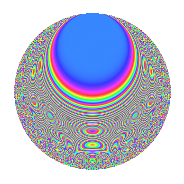# Properties

 Label 6001.2.a.aLevel 6001 Weight 2 Character orbit 6001.a Self dual Yes Analytic conductor 47.918 Analytic rank 1 Dimension 113 CM No

# Related objects

## Newspace parameters

 Level: $$N$$ = $$6001 = 17 \cdot 353$$ Weight: $$k$$ = $$2$$ Character orbit: $$[\chi]$$ = 6001.a (trivial)

## Newform invariants

 Self dual: Yes Analytic conductor: $$47.918226253$$ Analytic rank: $$1$$ Dimension: $$113$$ Fricke sign: $$1$$ Sato-Tate group: $\mathrm{SU}(2)$

## $q$-expansion

The dimension is sufficiently large that we do not compute an algebraic $$q$$-expansion, but we have computed the trace expansion.

 $$\operatorname{Tr}(f)(q) =$$ $$113q$$ $$\mathstrut -\mathstrut 11q^{2}$$ $$\mathstrut -\mathstrut 11q^{3}$$ $$\mathstrut +\mathstrut 103q^{4}$$ $$\mathstrut -\mathstrut 19q^{5}$$ $$\mathstrut -\mathstrut 13q^{6}$$ $$\mathstrut +\mathstrut 11q^{7}$$ $$\mathstrut -\mathstrut 36q^{8}$$ $$\mathstrut +\mathstrut 94q^{9}$$ $$\mathstrut +\mathstrut O(q^{10})$$ $$\operatorname{Tr}(f)(q) =$$ $$113q$$ $$\mathstrut -\mathstrut 11q^{2}$$ $$\mathstrut -\mathstrut 11q^{3}$$ $$\mathstrut +\mathstrut 103q^{4}$$ $$\mathstrut -\mathstrut 19q^{5}$$ $$\mathstrut -\mathstrut 13q^{6}$$ $$\mathstrut +\mathstrut 11q^{7}$$ $$\mathstrut -\mathstrut 36q^{8}$$ $$\mathstrut +\mathstrut 94q^{9}$$ $$\mathstrut -\mathstrut 5q^{10}$$ $$\mathstrut -\mathstrut 40q^{11}$$ $$\mathstrut -\mathstrut 19q^{12}$$ $$\mathstrut -\mathstrut 18q^{13}$$ $$\mathstrut -\mathstrut 48q^{14}$$ $$\mathstrut -\mathstrut 63q^{15}$$ $$\mathstrut +\mathstrut 79q^{16}$$ $$\mathstrut -\mathstrut 113q^{17}$$ $$\mathstrut -\mathstrut 32q^{18}$$ $$\mathstrut -\mathstrut 46q^{19}$$ $$\mathstrut -\mathstrut 56q^{20}$$ $$\mathstrut -\mathstrut 46q^{21}$$ $$\mathstrut +\mathstrut 14q^{22}$$ $$\mathstrut -\mathstrut 35q^{23}$$ $$\mathstrut -\mathstrut 42q^{24}$$ $$\mathstrut +\mathstrut 88q^{25}$$ $$\mathstrut -\mathstrut 89q^{26}$$ $$\mathstrut -\mathstrut 41q^{27}$$ $$\mathstrut +\mathstrut 20q^{28}$$ $$\mathstrut -\mathstrut 51q^{29}$$ $$\mathstrut -\mathstrut 18q^{30}$$ $$\mathstrut -\mathstrut 57q^{31}$$ $$\mathstrut -\mathstrut 93q^{32}$$ $$\mathstrut -\mathstrut 40q^{33}$$ $$\mathstrut +\mathstrut 11q^{34}$$ $$\mathstrut -\mathstrut 69q^{35}$$ $$\mathstrut +\mathstrut 18q^{36}$$ $$\mathstrut +\mathstrut 16q^{37}$$ $$\mathstrut -\mathstrut 74q^{38}$$ $$\mathstrut -\mathstrut 51q^{39}$$ $$\mathstrut +\mathstrut 2q^{40}$$ $$\mathstrut -\mathstrut 87q^{41}$$ $$\mathstrut -\mathstrut 23q^{42}$$ $$\mathstrut -\mathstrut 32q^{43}$$ $$\mathstrut -\mathstrut 110q^{44}$$ $$\mathstrut -\mathstrut 17q^{45}$$ $$\mathstrut -\mathstrut 17q^{46}$$ $$\mathstrut -\mathstrut 161q^{47}$$ $$\mathstrut -\mathstrut 36q^{48}$$ $$\mathstrut +\mathstrut 56q^{49}$$ $$\mathstrut -\mathstrut 69q^{50}$$ $$\mathstrut +\mathstrut 11q^{51}$$ $$\mathstrut -\mathstrut 49q^{52}$$ $$\mathstrut -\mathstrut 48q^{53}$$ $$\mathstrut -\mathstrut 38q^{54}$$ $$\mathstrut -\mathstrut 79q^{55}$$ $$\mathstrut -\mathstrut 171q^{56}$$ $$\mathstrut +\mathstrut 20q^{57}$$ $$\mathstrut +\mathstrut 13q^{58}$$ $$\mathstrut -\mathstrut 174q^{59}$$ $$\mathstrut -\mathstrut 146q^{60}$$ $$\mathstrut -\mathstrut 34q^{61}$$ $$\mathstrut -\mathstrut 34q^{62}$$ $$\mathstrut -\mathstrut 14q^{63}$$ $$\mathstrut +\mathstrut 62q^{64}$$ $$\mathstrut -\mathstrut 22q^{65}$$ $$\mathstrut -\mathstrut 60q^{66}$$ $$\mathstrut -\mathstrut 50q^{67}$$ $$\mathstrut -\mathstrut 103q^{68}$$ $$\mathstrut -\mathstrut 59q^{69}$$ $$\mathstrut -\mathstrut 58q^{70}$$ $$\mathstrut -\mathstrut 189q^{71}$$ $$\mathstrut -\mathstrut 123q^{72}$$ $$\mathstrut -\mathstrut 4q^{73}$$ $$\mathstrut -\mathstrut 24q^{74}$$ $$\mathstrut -\mathstrut 106q^{75}$$ $$\mathstrut -\mathstrut 92q^{76}$$ $$\mathstrut -\mathstrut 78q^{77}$$ $$\mathstrut -\mathstrut 42q^{78}$$ $$\mathstrut +\mathstrut 8q^{79}$$ $$\mathstrut -\mathstrut 150q^{80}$$ $$\mathstrut +\mathstrut 13q^{81}$$ $$\mathstrut +\mathstrut 6q^{82}$$ $$\mathstrut -\mathstrut 109q^{83}$$ $$\mathstrut -\mathstrut 114q^{84}$$ $$\mathstrut +\mathstrut 19q^{85}$$ $$\mathstrut -\mathstrut 116q^{86}$$ $$\mathstrut -\mathstrut 106q^{87}$$ $$\mathstrut +\mathstrut 54q^{88}$$ $$\mathstrut -\mathstrut 170q^{89}$$ $$\mathstrut -\mathstrut q^{90}$$ $$\mathstrut -\mathstrut 43q^{91}$$ $$\mathstrut -\mathstrut 94q^{92}$$ $$\mathstrut -\mathstrut 69q^{93}$$ $$\mathstrut -\mathstrut 35q^{94}$$ $$\mathstrut -\mathstrut 78q^{95}$$ $$\mathstrut -\mathstrut 44q^{96}$$ $$\mathstrut -\mathstrut 3q^{97}$$ $$\mathstrut -\mathstrut 68q^{98}$$ $$\mathstrut -\mathstrut 119q^{99}$$ $$\mathstrut +\mathstrut O(q^{100})$$

## Embeddings

For each embedding $$\iota_m$$ of the coefficient field, the values $$\iota_m(a_n)$$ are shown below.

For more information on an embedded modular form you can click on its label.

Label $$a_{2}$$ $$a_{3}$$ $$a_{4}$$ $$a_{5}$$ $$a_{6}$$ $$a_{7}$$ $$a_{8}$$ $$a_{9}$$ $$a_{10}$$
1.1 −2.78095 −2.69588 5.73371 2.30800 7.49712 4.50681 −10.3833 4.26777 −6.41845
1.2 −2.76448 −0.301476 5.64235 −1.88471 0.833425 2.57260 −10.0692 −2.90911 5.21024
1.3 −2.72078 −0.920111 5.40267 −2.98260 2.50342 −1.43517 −9.25792 −2.15340 8.11501
1.4 −2.69808 2.45523 5.27961 −1.37053 −6.62441 5.08280 −8.84865 3.02818 3.69780
1.5 −2.64384 −0.876989 4.98991 2.40505 2.31862 1.36446 −7.90487 −2.23089 −6.35858
1.6 −2.63611 2.68277 4.94905 −3.99469 −7.07208 −3.60242 −7.77401 4.19728 10.5304
1.7 −2.63080 1.97535 4.92113 2.13953 −5.19675 1.65541 −7.68492 0.902000 −5.62867
1.8 −2.58651 −0.844986 4.69006 −0.771482 2.18557 −2.98561 −6.95787 −2.28600 1.99545
1.9 −2.50694 0.525806 4.28474 −1.87738 −1.31816 4.48031 −5.72770 −2.72353 4.70647
1.10 −2.49624 1.48745 4.23122 −3.58200 −3.71302 1.79069 −5.56966 −0.787505 8.94153
1.11 −2.49146 −2.38691 4.20738 −2.02282 5.94689 1.32918 −5.49960 2.69734 5.03978
1.12 −2.49070 2.79631 4.20359 2.44678 −6.96477 −1.91297 −5.48850 4.81934 −6.09421
1.13 −2.30912 −2.56184 3.33205 −3.64906 5.91560 −1.49745 −3.07586 3.56301 8.42614
1.14 −2.26295 −1.98147 3.12095 3.45141 4.48397 −1.45595 −2.53665 0.926221 −7.81036
1.15 −2.22225 −0.120604 2.93840 1.74504 0.268012 −3.61581 −2.08537 −2.98545 −3.87792
1.16 −2.20707 2.35834 2.87116 2.79748 −5.20501 −1.23289 −1.92271 2.56174 −6.17423
1.17 −2.18121 0.0139647 2.75766 −0.236603 −0.0304599 2.78463 −1.65261 −2.99980 0.516079
1.18 −2.15299 2.37258 2.63537 −0.231510 −5.10813 3.32403 −1.36794 2.62912 0.498439
1.19 −2.14256 −2.11238 2.59055 1.25903 4.52589 1.44603 −1.26528 1.46215 −2.69753
1.20 −2.09226 2.92082 2.37755 −1.12068 −6.11112 −1.50686 −0.789928 5.53120 2.34475
See next 80 embeddings (of 113 total)
 $$n$$: e.g. 2-40 or 990-1000 Embeddings: e.g. 1-3 or 1.113 Significant digits: Format: Complex embeddings Normalized embeddings Satake parameters Satake angles

## Inner twists

This newform does not have CM; other inner twists have not been computed.

## Atkin-Lehner signs

$$p$$ Sign
$$17$$ $$1$$
$$353$$ $$1$$